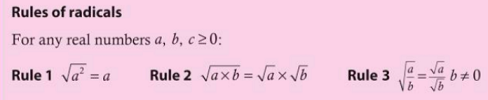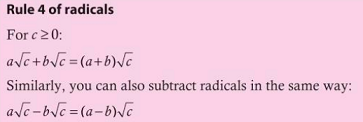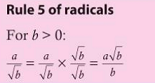MathHW.net

### 9 IP Math Units

1. Representing quantities within data sets
4.1, 4.2, 6.2
2. Generalizing different representations to make predictions
4.3, 12.3
3. Equality Models
11.1, 14.3
4. Simplifying is a change for the better
5.1, 10.3
5. Measuring and reasoning quantitatively
6.1, 7.1, 7.2
6. Equivalent forms of quadratic equations
8.2, 9.2, 11.2
7. Exploring patters and generalizing solutions
8.1, 12.1, 12.2

# Unit 4

## 10.3 Getting more done in less time

• F Direct and inverse variatio
• How do you find a constant of proportionality?
• What does a proportional relationship look like?

File List
• 10.3 F.docx
• What you should already know how to do
• Exploration 1 (selling light bulbs)
• Reflect and discuss 1
• Reflect and discuss 2
• Example 1
• Reflect and discuss 3
• Example 2
• Practice 1 (6 problems; #5 and #6 are Problem Solving)
• 10.3 F Part 2
• Exoloration 2
• Reflect and discuss 4
• Example 3
• Practice 2 (9 problems; #6-9 are Problem Solving)

• C Different types of variation
• What does in mean to be proportional?
• How does changing one variable in a proportional relationship affect the other?

File List
• 10-3-C Different types of Variation
• Exploration 3 (circle, radius r)
• Exploration 4 (wall tiles)
• Reflect and discuss 5
• Exploration 5 (looking a equations, such as y = kx
• Example 4
• Example 5
• Reflect and discuss 6
• Practice 3 (9 problems; #5 - #8 are Problem Solving)
• Exploration 6 (introduces exponents)
• Reflect and discuss 7
• Practice 4 (3 problems; #2 - #3 are Problem Solving)
• 10-3-C Different Types of Variation - Key

• 10-3-D Modeling Proportional Relationships
• Can situations seem proportional when they are not?
• Is simpler always better?

File List
• 10-3-D Modeling Proportional Relationships
• Exploration 7 (coffee shop)
• Exploration 8 (sketching graphs)
• Reflect and discuss 8
• Section 10-3 Summary
• Section 10-3 Mixed Practice problems (13 problems; 8-13 are Problem Solving)
• Section 10-3 Review in Context (6 problems)
• Section 10-3 Reflect and discuss
• 10-3-D Table (contains a table to make one of the questions easier to answer)
• 10-3-D Modeling Proportional Relationships Key
• Summary

• Assessments
• Skill quizzes / Test

• IXL Activities
Algebra 1
• R.1 Identify proportiona relationships
• R.2 Find the constant of variation
• R.3 Graph a proportional relationship
• R.4 Write direct variation equations
• R.5 Write and solve direct variation equations
• R.6 Identify direct variation and inverse variation
• R.7 Write inverse variation equations
• R.8 Write and solve inverse variation equations

## 5.1 Are you saying I'm irrational?

• Introduction

File Introduction to Unit - 4 - Google Slides view as a presentation

• Objectivs and Inquiry Questions
• Prior Learning / prerequisite skills

$\sqrt{2}$
• Exploration 1
• Reflect and discuss 1
• Exploration 2
• Practice 1
• Exploration 3
• Reflect and discuss 2

• Exploration 4
• Example 1• Practice 2
• Exploration 5
• Reflect and discuss 3
• Exploration 6
• Example 2
• Reflect and discuss 4
• Example 3
• Practice 3
• Exploration 7
• Example 4
• Reflect and discuss 5• Practice 4
• Reflect and discuss 6
• Exploration 8
• Reflect and discuss 7• Example 5
• Example 6
• Practice 5

• Objective B
• Exploration 9

• Summary
• Mixed Practice

• Assessments
• Skill quizzes - one five point quiz on each of the five rules
• 30 - 40 point test with a mix of questions similar to the Mixed Practice problems. Students that complete the practice problems and IXL activities that are assigned will do well on this test which will probably be unannounced. As an unannounced assessment this can be retaken as needed to demonstrate proficiency with radicals (surds).

• IXL Activities

## E5.1 Are you saying I'm irrational?

• Introduction

File Introduction to Unit - 4 - Google Slides view as a presentation

• Objectivs and Inquiry Questions
• Prior Learning / prerequisite skills

$\sqrt{2}$
• Exploration 1
• Reflect and discuss 1
• Exploration 2
• Practice 1
• Exploration 3
• Reflect and discuss 2

• Exploration 4
• Example 1• Practice 2
• Exploration 5
• Reflect and discuss 3
• Exploration 6
• Example 2
• Reflect and discuss 4
• Example 3
• Practice 3
• Exploration 7
• Example 4
• Reflect and discuss 5• Practice 4
• Reflect and discuss 6
• Exploration 8
• Reflect and discuss 7• Example 5
• Example 6
• Practice 5